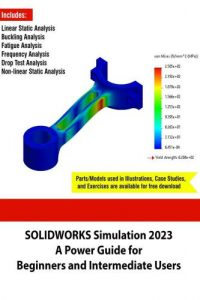اسم المؤلف
التاريخ
13 أغسطس 2023
المشاهدات
238
التقييمLoading...

SOLIDWORKS Simulation 2023 – A Power Guide for Beginners and Intermediate Users
Chapter 1, “Introduction to FEA and SOLIDWORKS Simulation,”
introduces SOLIDWORKS Simulation, various types of analysis,
introduction to Finite Element Analysis (FEA), and different phases
of Finite Element Analysis (FEA): Pre-processing, Solution, andPost-processing. It also explains various terms and definitions used
in Finite Element Analysis (FEA) in addition to different types of
elements, the application areas of FEA, system requirements for
installing SOLIDWORKS Simulation, and SOLIDWORKS interface.
Besides, this chapter discusses how to invoke different
Simulation.
Chapter 2, “Introduction to Analysis Tools and Static Analysis,” introduces
various assumptions for considering the linear static analysis
chapter also explains how to define the analysis unit and material
properties for geometry, adding a new material and customizing the
material properties. Besides, it introduces boundary conditions
(fixtures and loads), meshing geometry, and identifying poor quality
mesh elements.
Chapter 3, “Case Studies of Static Analysis,” discusses various case
studies of linear static analysis: Static Analysis of a Rectangular
Plate, Static Analysis of a Bracket with Mesh Control, Static Analysis
of a Symmetrical Model, Static Analysis of a Torispherical Head with
Shell Elements, and Static Analysis of a Weldment Frame with Beam
Elements.
Chapter 4, “Interactions and Connectors,” discusses various interactions
and connectors available in SOLIDWORKS Simulation. It introduces
how to perform the static analysis of various case studies having
interaction problems: Static Analysis of a Hook Assembly with
Interactions, Static Analysis of a Flange Assembly with Bolt
Connectors, and Static Analysis of an Assembly with Edge WeldConnectors.
analysis with them.
Chapter 6, “Buckling Analysis,” introduces the concept of the buckling
analysis and how to perform the buckling analysis of different case
studies: Buckling Analysis of a Pipe Support, Buckling Analysis of a
Beam.
Chapter 7, “Fatigue Analysis,” discusses about the failure of a design
due to fatigue when the design undergoes cyclic loads. It also
introduces how to perform the fatigue analysis.
Chapter 8, “Frequency Analysis,” introduces how to perform the
frequency analysis to calculate the natural/resonant frequencies, the
mode shapes associated to each natural frequency, and the mass
participations in X, Y, and Z directions.
Chapter 9, “Drop Test Analysis,” introduces how to perform the drop
test analysis to calculate the impact of a part or an assembly with a
rigid or flexible floor.
Chapter 10, “Non-Linear Static Analysis,” introduces various
assumptions for considering the non-linear static analysis problems.
It discusses different iterative methods (Newton-Raphson (NR)
scheme and Modified Newton-Raphson (MNR) scheme) and
incremental methods (Force, Displacement, and Arc Length) to find
the equilibrium solutions for the non-linear analysis. The chapter also
discusses different types of non-linearities (material non-linearities,
geometric non-linearities, and contact non-linearities) and how toperform the non-linear analysis of various case studies: Non-Linear
Static Analysis of a Shackle, Non-Linear Static Analysis of a Handrail
Clamp Assembly, and Non-Linear Static Analysis of a CantileverBeam.

كلمة سر فك الضغط : books-world.net